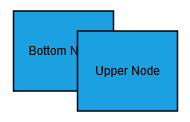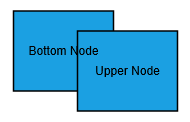# Label

23 Sep 202018 minutes to read

Label is a block of text that can be displayed over a node or connector. Label is used to textually represent an object with a string that can be edited at run time. You can add Multiple Labels to a node/connector.

## Create Label

You can add a label to a node/connector by defining the label object and adding that to the `Labels` collection of node/connector. The `Text` property of label defines the text to be displayed. The following code illustrates how to create a Label.

• RAZOR
• ``````@*Initializes diagram control*@
<div>
<ej-diagram id="diagram" width="900px" height="600px"></ej-diagram>
</div>``````
• RAZOR
• ``````<ej-diagram id="diagram" width="900px" height="600px">
<e-nodes>
<e-node name="node1" width="100" height="100" offset-X="100" offset-Y="100" fill-color="#1BA0E2" border-color="black">
<e-labels>
<e-diagram-label text="Label"></e-diagram-label>
</e-labels>
</e-node>
</e-nodes>
<e-connectors>
<e-connector>
<e-source-point x="200" y="50"></e-source-point>
<e-target-point x="300" y="150"></e-target-point>
<e-segments>
<e-segment type="Orthogonal" direction="bottom" length="50"></e-segment>
</e-segments>
<e-labels>
<e-diagram-label text="Label" fill-color="white"></e-diagram-label>
</e-labels>
</e-connector>
</e-connectors>
</ej-diagram>``````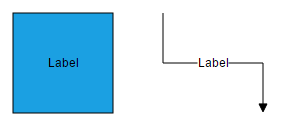To explore more label properties, refer to Label Properties.

## Update Label at runtime

The client side API `updateLabel` is used to update the labels at run time.

The following code example illustrates how to change the label properties.

• C#
• ``````var diagram = \$("#diagram").ejDiagram("instance");
var selectedObject = diagram.model.selectedItems.children;
diagram.updateLabel(selectedObject.name, selectedObject.labels, { text: "label", fillColor: "red" });``````

## Alignment

Label can be aligned relative to the node boundaries. It has margin, offset, horizontal and vertical alignment settings. It is quite tricky when all four alignments are used together but gives you more control over alignment.

### Offset

The `Offset` property of label is used to align the labels based on fractions. 0 represents top/left corner, 1 represents bottom/right corner, and 0.5 represents half of width/height.

The following image shows the relationship between the label position (black colored circle) and offset (fraction values).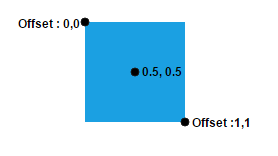### Horizontal and vertical alignments

The `HorizontalAlignment` property of label is used to set how the label is horizontally aligned at the label position determined from the fraction values. The `VerticalAlignment` property is used to set how label is vertically aligned at the label position.

The following tables illustrates all the possible alignments visually with offset (0, 0).

Horizontal Alignment Vertical Alignment Output with Offset(0,0)
Left Top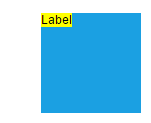Center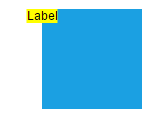Right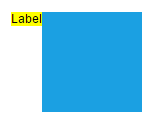Left Center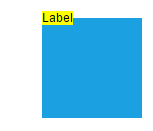Center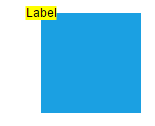Right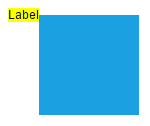Left Bottom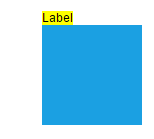Center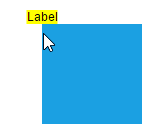Right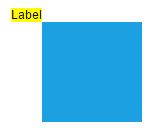The following codes illustrates how to align labels.

• RAZOR
• ``````<ej-diagram id="diagram" width="900px" height="600px">
<e-nodes>
<e-node name="node1" width="100" height="100" offset-X="100" offset-Y="100" fill-color="#1BA0E2" border-color="black">
<e-labels>
<e-diagram-label text="Label" horizontal-alignment="Left" vertical-alignment="Center" text-align="Center">
<e-offset x="0" y="0.5f"></e-offset>
</e-diagram-label>
</e-labels>
</e-node>
</e-nodes>
</ej-diagram>``````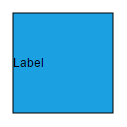### Margin

Margin is an absolute value used to add some blank space in any one of its four sides. You can displace the labels with the `Margin` property.
The following code example illustrates how to align a label based on its `Offset`, `HorizontalAlignment`, `VerticalAlignment` and `Margin` values.

• RAZOR
• ``````<ej-diagram id="diagram" width="900px" height="600px">
<e-nodes>
<e-node name="node1" width="100" height="100" offset-X="100" offset-Y="100" fill-color="#1BA0E2" border-color="black">
<e-labels>
<e-diagram-label text="Label" horizontal-alignment="Center" vertical-alignment="Top">
<e-offset x="0.5f" y="1"></e-offset>
<e-margin top="10"></e-margin>
</e-diagram-label>
</e-labels>
</e-node>
</e-nodes>
</ej-diagram>``````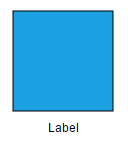### Text Alignment

The `TextAlign` property of label allows you to set how the text should be aligned (left, right, center, or justify) inside the text block. The following codes illustrate how to set textAlign for a label.

• RAZOR
• ``````<ej-diagram id="diagram" width="900px" height="600px">
<e-nodes>
<e-node name="node1" width="100" height="100" offset-X="100" offset-Y="100" fill-color="#1BA0E2" border-color="black">
<e-labels>
<e-diagram-label text="Text Align is set asLeft" text-align="Left"></e-diagram-label>
</e-labels>
</e-node>
</e-nodes>
</ej-diagram>``````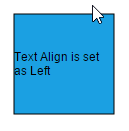TextAlign Image
LeftRight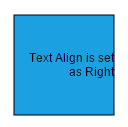Center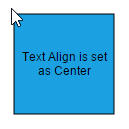Justify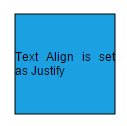## Wrapping

When text overflows node boundaries, you can control it by using text wrapping. So, it is wrapped into multiple lines. The `Wrapping` property of label defines how the text should be wrapped. The following code illustrates how to wrap a text in a node.

• RAZOR
• ``````<ej-diagram id="diagram" width="900px" height="600px">
<e-nodes>
<e-node name="node1" width="100" height="100" offset-X="100" offset-Y="100" fill-color="#1BA0E2" border-color="black">
<e-labels>
<e-diagram-label text="Label Text Wrapping" wrapping="Wrap"></e-diagram-label>
</e-labels>
</e-node>
</e-nodes>
</ej-diagram>``````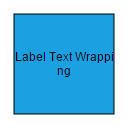Values Description Image
NoWrap Text will not be wrapped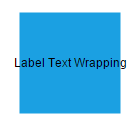Wrap Text-wrapping occurs when the text overflows beyond the available node width.WrapWithOverflow (Default) Text-wrapping occurs when the text overflows beyond the available node width. However, the text may overflow beyond the node width in the case of a very long word.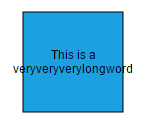## Appearance

You can change the font style of the labels with the font specific properties(`FontSize`,`FontFamily`,`FontColor`.,). The following code illustrates how to customize the appearance of a label.

• RAZOR
• ``````<ej-diagram id="diagram" width="900px" height="600px">
<e-nodes>
<e-node name="node1" width="100" height="100" offset-X="100" offset-Y="100" fill-color="#1BA0E2" border-color="black">
<e-labels>
<e-diagram-label text="Label Text" font-size="12" font-family="TimesNewRoman" font-color="black" bold="true" italic="true" text-decoration="Underline"></e-diagram-label>
</e-labels>
</e-node>
</e-nodes>
</ej-diagram>``````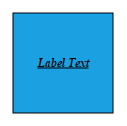The fill and border appearances of the text can also be customized with appearance specific properties of label.The following code illustrates how to customize background and border of a label.

• RAZOR
• ``````<ej-diagram id="diagram" width="900px" height="600px">
<e-nodes>
<e-node name="node1" width="100" height="100" offset-X="100" offset-Y="100" fill-color="#1BA0E2" border-color="black">
<e-labels>
<e-diagram-label text="Label Text" fill-color="white" border-color="black" border-width="1"></e-diagram-label>
</e-labels>
</e-node>
</e-nodes>
</ej-diagram>``````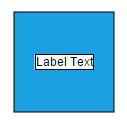## Drag

A Label can be displaced from its original position to any preferred location interactively. Dragging is disabled by default. You can enable label dragging with the `Constraints` property of node/connector. The following code illustrates how to enable label dragging.

• RAZOR
• ``````<ej-diagram id="diagram" width="900px" height="600px">
<e-nodes>
<e-node name="node1" width="100" height="100" offset-X="100" offset-Y="100" fill-color="#1BA0E2" border-color="black" constraints="Default|NodeConstraints.DragLabel">
<e-labels>
<e-diagram-label text="Label"></e-diagram-label>
</e-labels>
</e-node>
</e-nodes>
</ej-diagram>

<ej-diagram id="diagram" width="900px" height="600px">
<e-connectors>
<e-connector constraints="Default|ConnectorConstraints.DragLabel">
<e-source-point x="200" y="50"></e-source-point>
<e-target-point x="300" y="150"></e-target-point>
<e-segments>
<e-segment type="Orthogonal" direction="bottom" length="50"></e-segment>
</e-segments>
<e-labels>
<e-diagram-label text="Label" fill-color="white"></e-diagram-label>
</e-labels>
</e-connector>
</e-connectors>
</ej-diagram>``````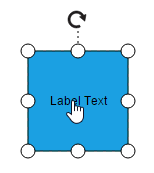## Rotate

You can rotate the labels to any desired angle. Labels are rotated to the angle that is defined by the `RotateAngle` property of label. The following code illustrates how to rotate a label.

• RAZOR
• ``````<ej-diagram id="diagram" width="900px" height="600px">
<e-nodes>
<e-node name="node1" width="100" height="100" offset-X="100" offset-Y="100" fill-color="#1BA0E2" border-color="black">
<e-labels>
<e-diagram-label text="Label Text" rotate-angle="45"></e-diagram-label>
</e-labels>
</e-node>
</e-nodes>
</ej-diagram>``````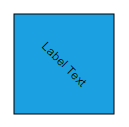NOTE

There is no built-in support to rotate labels interactively.

## Edit

Diagram provides support to edit a Label at runtime, either programmatically or interactively. By default, label is in View mode. But it can be brought to edit mode in two ways;

1. By double-clicking the label.
2. By selecting the item and pressing the F2 key.

Double-clicking any label will enables editing of that. Double-clicking the node enables first label editing. When the focus of editor is lost, the label for the node is updated.

You can programmatically edit the label by changing the `Mode` of the label. The following code illustrates how to edit the label programmatically.

• RAZOR
• ``````var diagram = \$("#diagram").ejDiagram("instance");
var node = diagram.model.selectedItems.children;
//Sets label mode as Edit
var options = {
mode: ej.datavisualization.Diagram.LabelEditMode.Edit
};
diagram.updateLabel(node.name, node.labels, options);``````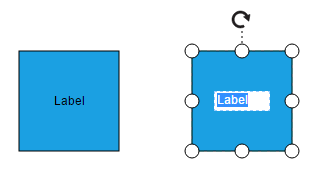Diagram allows to create read only labels. You have to set the `ReadOnly` property of label to enable/disable the read only mode. The following code illustrates how to enable readOnly mode.

• RAZOR
• ``````<ej-diagram id="diagram" width="900px" height="600px">
<e-nodes>
<e-node name="node1" width="100" height="100" offset-X="100" offset-Y="100" fill-color="#1BA0E2" border-color="black">
<e-labels>
</e-labels>
</e-node>
</e-nodes>
</ej-diagram>``````

### Drag Limit

Diagram label dragging can be restricted by enabling/disabling `DragLimit` constraints of the connectors. Also we can define the drag limit in all direction using `DragLimit` property of the connector. Drag limit only applicable for connector labels.

• RAZOR
• ``````<ej-diagram id="diagram" width="1000px" height="600px" enable-context-menu="false">
<e-connectors>
@* Enable DragLimit constraints to the connector. *@
<e-connector name="Connector4" constraints="Default|ConnectorConstraints.DragLabel|ConnectorConstraints.DragLimit">
<e-labels>
<e-diagram-label  text="Meeting with board" font-color="White">
@* Defines the drag limit property in all direction. *@
<e-drag-limit left="30" right="30" bottom="30" top="30"></e-drag-limit>
</e-diagram-label>
</e-labels>
</e-connector>
</e-connectors>
</ej-diagram>``````

## Multiple labels

You can add any number of labels to a node or connector. The following code illustrates how to add multiple labels to a node.

• RAZOR
• ``````<ej-diagram id="diagram" width="900px" height="600px">
<e-nodes>
<e-node name="node1" width="100" height="100" offset-X="100" offset-Y="100" fill-color="#1BA0E2" border-color="black">
<e-labels>
<e-diagram-label text="Left" font-color="white">
<e-offset x="0.12f" y="0.1f"></e-offset>
</e-diagram-label>
<e-diagram-label text="Center" font-color="white">
<e-offset x="0.5f" y="0.5f"></e-offset>
</e-diagram-label>
<e-diagram-label text="Right" font-color="white">
<e-offset x="0.82f" y="0.9f"></e-offset>
</e-diagram-label>
</e-labels>
</e-node>
</e-nodes>
</ej-diagram>``````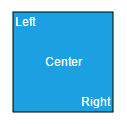## Limitation

• To enable faster rendering, labels are rendered in a separate layer because of this, all the labels always stay on top. When two nodes are overlapped, text of underlying node is not hidden by the overlapped node.
Expected behavior Current behavior